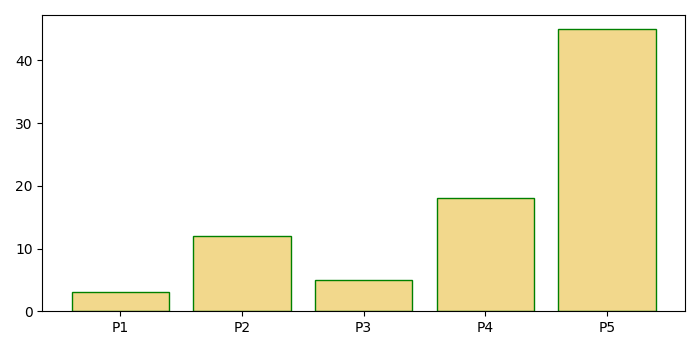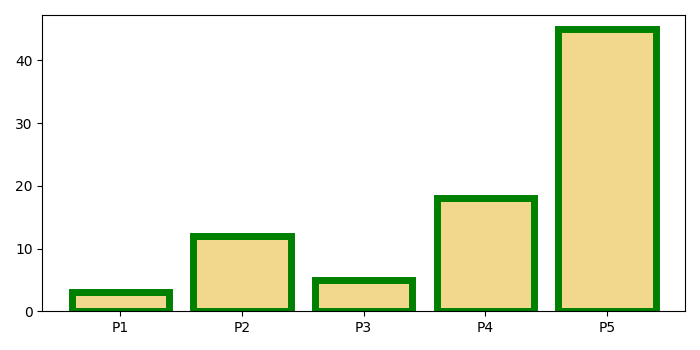# How to control the border of a bar patch in matplotlib?

MatplotlibPythonData Visualization

To control the border of a bar patch in matplotlib, we can take the following steps

## Steps

• Set the figure size and adjust the padding between and around the subplots.

• Create a list of heights and a tuple for labels.

• Use the bar() method with edgecolor in the argument to control the color of the bar patch. Here we have used edgecolor='green'.

• Set the ticks and labels of the X-axis.

• To display the figure, use Show() method.

## Example

import numpy as np
import matplotlib.pyplot as plt

plt.rcParams["figure.figsize"] = [7.00, 3.50]
plt.rcParams["figure.autolayout"] = True

height = [3, 12, 5, 18, 45]
labels = ('P1', 'P2', 'P3', 'P4', 'P5')

x_pos = np.arange(len(labels))

plt.bar(x_pos, height, color=(0.9, 0.7, 0.1, 0.5), edgecolor='green')
plt.xticks(x_pos, labels)

plt.show()


## Output

It will produce the following output −You can change the width of the borders by introducing the linewidth parameter in the bar() method. Suppose we take linewidth=5, then It will produce the following output −